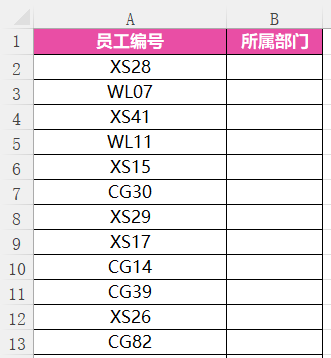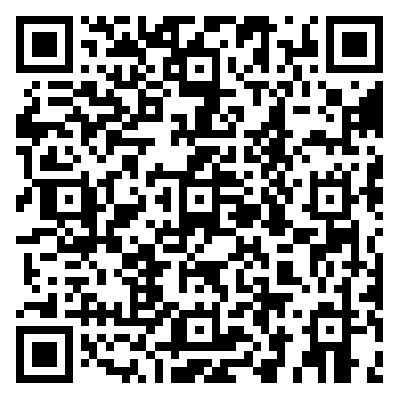# Excel教程：IF函数的多层嵌套工作案例分享

[日期：2022-12-24]   来源：IT部落窝  作者：IT部落窝   阅读：3[字体： ]

Excel中IF函数的单一用法，相信大家都明白。今天着重给大家分享IF函数的多层嵌套。学会之后，你就会明白在if函数中再嵌一个if函数怎么做。B2单元格公式为：=IF(LEFT(A2,2)="XS","销售部",IF(LEFT(A2,2)="CG","采购部","物流部"))

=IFS(LEFT(A2,2)="XS","销售部",LEFT(A2,2)="CG","采购部",LEFT(A2,2)="WL","物流部")分享到： QQ空间 新浪微博 腾讯微博 人人网